# Collaborative filtering with FastAIA collaborative filtering model/recommendation system seeks to predict the rating or preference a user would give to an item given his old item ratings or preferences. Recommendation systems are used by pretty much every major company in order to enhance the quality of their services.

In one of my first articles, I created a book recommendation system using Keras a high-level API build on Tensorflow. In this article, I will show you how to build the same recommendation system using the FastAI library as well as how to build a neural network based model to get even better results.

For our data, we will use the goodbooks-10k dataset which contains ten thousand different books and about one million ratings. It has three features the book_id, user_id and rating. If you want you can get the all the data files as well as the complete code covered in this article from my Github repository.

If you prefer a visual tutorial you can check out my FastAi videos.

### Getting data

After downloading the data-set from Kaggle we need to load in FastAIs collab filtering module, specify the path to the data-set and load in the csv containing the ratings as well as the csv containing the book information.

from fastai.collab import *# specify pathpath = Path('<path to the data-set>')print(path.ls())# load in ratings dataratings = pd.read_csv(path/'ratings.csv')print(ratings.head())# load in book information databooks = pd.read_csv(path/'books.csv')print(books.head())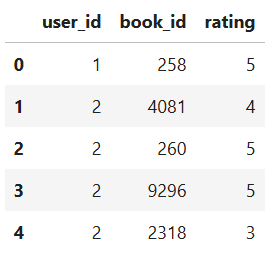Figure 2: Recommendation dataframe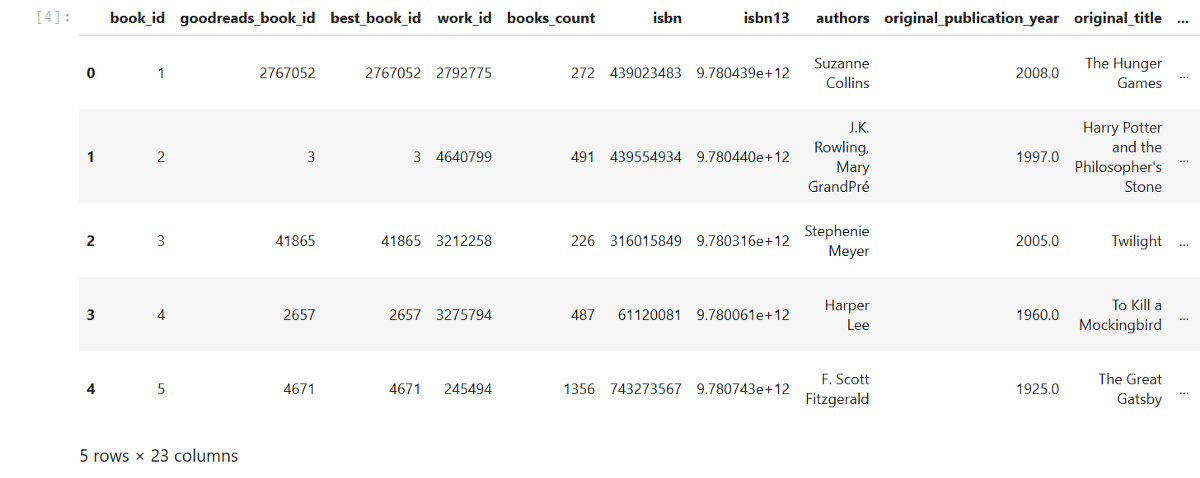Figure 3: Book information dataframe

With our data loaded in we can create a CollabDataBunch, which is a databunch specifically created for collaborative filtering problems. We will pass it our rating data, a random seed as well as the size of our validation set which is defined by the valid_pct argument.

data = CollabDataBunch.from_df(ratings, seed=42, valid_pct=0.1, user_name='user_id', item_name='book_id', rating_name='rating')

As always we can show a batch of our data using the show_batch method.

data.show_batch()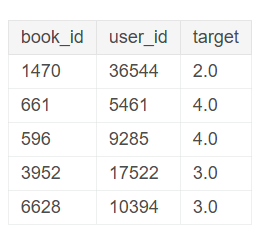Figure 4: Random data batch

The last thing we need to do before creating and training our model is getting the max and min values of our ratings. We will then pass these values to our model so it can then squeeze the final outputs between these two values.

ratings.rating.min(), ratings.rating.max()Output: (1, 5)

### EmbeddingDotBias Model

FastAI provides two different types of collab models. A simple model called EmbeddingDotBias which used for almost all recommendation systems a few years ago. It creates embeddings for both users and books and then takes the dot product of them. The second is a neural network based model which uses embeddings and fully-connected layers.

An embedding is a mapping from discrete objects, such as words or ids of books and users in our case, to a vector of continuous values. This can be used to find similarities between the discrete objects, that wouldn’t be apparent to the model if it didn’t use embedding layers.

These embedding vectors are low-dimensional and get updated whilst training the network.

Both models can be created using the collab_learner class. Standardly the use_nn argument is set to false and therefore we are creating an EmbeddingDotBias model.

As further arguments, we can pass the collab learner the n_factors argument which represents the size of the embedding vectors as well as the yrange argument which specifies the range of the rating values we found earlier.

learn = collab_learner(data, n_factors=40, y_range=(1, 5), wd=1e-1)

Now we can find the learning rate, train our model using the fit_one_cycle method and save the model. If you aren’t familiar with this process yet I would recommend you to check out my first article about Image Classification using the FastAI library.

learn.lr_find() # find learning ratelearn.recorder.plot() # plot learning rate graph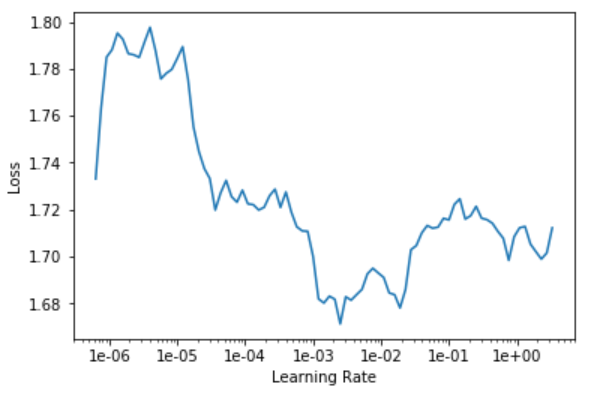Figure 5: Learning rate plot

learn.fit_one_cycle(5, 3e-4)Figure 6: Training results

learn.save('goodbooks-dot-1')

### EmbeddingNN Model

The second type of collaborative filtering model provided by FastAI is called EmbeddingNN. It provides us with the ability to create embeddings with different sizes and feed them into a neural network.

FastAI also provides us with the ability to tweak the number of layers and their units.

learn = collab_learner(data, use_nn=True, emb_szs={'user_id': 40, 'book_id':40}, layers=[256, 128], y_range=(1, 5))

As always the next steps are to find the learning rate and train the model.

learn.lr_find() # find learning ratelearn.recorder.plot() # plot learning rate graph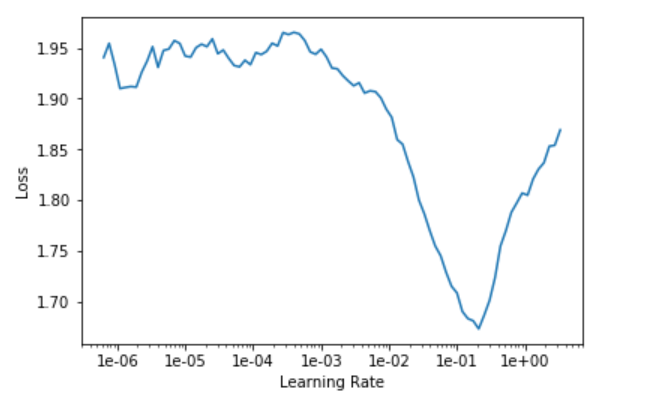Figure 7: Learning rate plot

learn.fit_one_cycle(5, 1e-2)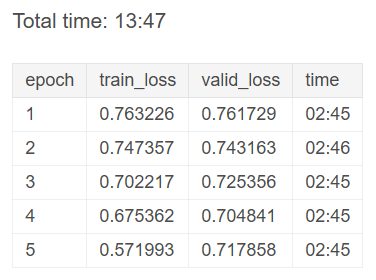Figure 8: Training results

We can see that the neural network performed a lot better than our dot-product model.

### Interpretation

Because the embeddings learned should represent the style and kind of books and users as best as possible they might contain interesting features which we can extract and visualize in order of getting insides.

For this purpose FastAI allows you to easily access both the user and book embeddings as well as their biases.

In this article, we will extract the book biases and weights in order to get insights of what books should be ranked lower or higher, using the embedding bias, as well as how similar some of the most popular books are using the embedding weights.

To start off we will load in our EmbeddingDotBias model and get the 1000 most popular books by how much reviews they have.

# load in EmbeddingDotBias modellearn = collab_learner(data, n_factors=40, y_range=(1, 5), wd=1e-1, model_dir="/tmp/model/", path="/tmp/")learn.load('goodbooks-dot-1');# get top booksg = ratings.groupby('book_id')['rating'].count()top_books = g.sort_values(ascending=False).index.values[:1000]top_books = top_books.astype(str)# create array containing the names of the top bookstop_books_with_name = []for book in top_books:    top_books_with_name.append(books[(books['id']==int(book))]['title'].iloc)    top_books_with_name = np.array(top_books_with_name)

Now we can extract the biases for the top books as well as the mean rating of the top books and print them out. With this, we can get information about the books that are generally rated low or high no matter what user is rating them.

# get biases for top booksbook_bias = learn.bias(top_books, is_item=True)# get mean ratingsmean_ratings = ratings.groupby('book_id')['rating'].mean()book_ratings = [(b, top_books_with_name[i], mean_ratings.loc[int(tb)]) for i, (tb, b) in enumerate(zip(top_books, book_bias))]# print book bias informationitem0 = lambda o:oprint(sorted(book_ratings, key=item0)[:15])print(sorted(book_ratings, key=item0, reverse=True)[:15])# get weightsbook_w = learn.weight(top_books, is_item=True)# transform weights to 3 dimensionsbook_pca = book_w.pca(3)# get prinicipal componentsfac0,fac1,fac2 = book_pca.t()book_comp = [(f, i) for f,i in zip(fac0, top_books_with_name)]# print fac0 informationprint(sorted(book_comp, key=itemgetter(0), reverse=True)[:10])print(sorted(book_comp, key=itemgetter(0))[:10])# print fac1 informationbook_comp = [(f, i) for f,i in zip(fac1, top_books_with_name)]print(sorted(book_comp, key=itemgetter(0), reverse=True)[:10])print(sorted(book_comp, key=itemgetter(0))[:10])Output:Top idx:array(['5000', '3315', '3313', '3312', '3311', '3309', '3308', '3307', '3306', '3304'], dtype='<U21')Top names:array(['Passion Unleashed (Demonica #3)', 'My Story', 'The Gargoyle', 'Pretty Baby', ...,       'Top Secret Twenty-One (Stephanie Plum, #21)', 'The Warrior Heir (The Heir Chronicles, #1)', 'Stone Soup',       'The Sixth Man (Sean King & Michelle Maxwell, #5)'], dtype='<U144')Most negative bias:[(tensor(-0.1021), 'The Almost Moon', 2.49), (tensor(-0.0341), 'Skinny Bitch', 2.9), (tensor(-0.0325), 'Bergdorf Blondes', 3.0), (tensor(-0.0316), 'The Particular Sadness of Lemon Cake', 2.93), (tensor(-0.0148), 'The Weird Sisters', 3.08)]...

We can also visualize the two principal components using a graphing library like Matplotlib.

idxs = np.random.choice(len(top_books_with_name), 50, replace=False)idxs = list(range(50))X = fac0[idxs]Y = fac2[idxs]plt.figure(figsize=(15,15))plt.scatter(X, Y)for i, x, y in zip(top_books_with_name[idxs], X, Y):    plt.text(x,y,i, color=np.random.rand(3)*0.7, fontsize=11)plt.show()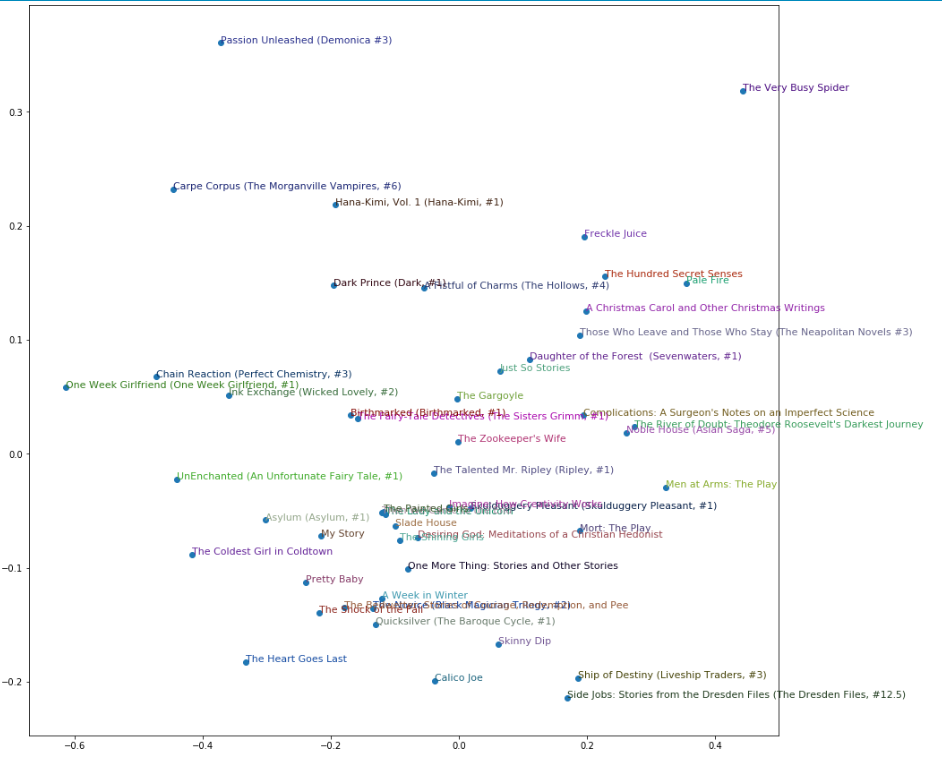Figure 9: Weight Embedding visualization

### Conclusion

A recommendation system seeks to predict the rating or preference a user would give to an item given his old item ratings or preferences.

The FastAI deep learning library provides us with functionality to easily load in our data and build our collaborative filtering/recommendation system model.

If you liked this article consider subscribing to my Youtube Channel and following me on social media.

The code covered in this article is available as a Github Repository.

If you have any questions, recommendations or critiques, I can be reached via Twitter or the comment section.